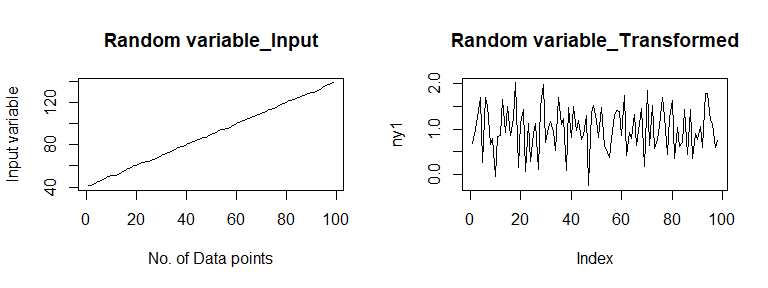# Demystifying UnitStat

#### 23-02-2021

What does Unit Stat do?

UnitStat helps the user’s understand whether the random variable under consideration is Stationary or Non-stationary using the Unit Root statistics (like ADF,PP, KPSS,ADF-GLS & NGP etc.) The function ensures to check all the pre-requisite and assumptions which are underlying the unit root test statistics (since the statistical software used by practitioners do not honour these assumptions) and return’s with a statement concluding whether the time series under consideration is stationary with “drift”, “drift & trend”, “trend”, “no drifts- no trend” or non-stationary. If the time series under consideration is observed to be non-stationary with a “drift” or “trend” or “both” component present, the function de-drifts and de-trends the series and performs the test again to produce the final output [see example’s below for detailed interpretation of the outputs].The function thus parses through all the output of all the 4 lags and produces an optimised interpretation of the results. Thus avoiding human error’s/misinterpretation of the results at the same time releasing the user from worrying about interpretation of the results. The function also plot’s the original as well as transformed input data for data visualization purposes.

How is UnitStat different from other test?

The interpretation of the stationary results are based on the 95% critical values only which are taken from Dicky and Fuller(1981). The function uses the original Dicky-Fuller test statistics as it was observed from Independent research that Unit roots are highly susceptible to produce incorrect results and are highly unstable at borderline. Along with Borderline instability, researchers have also pointed out the instability of these tests. Researchers have also pointed that, PP test performs worse than ADF in finite sample scenarios whereas KPSS is criticised for high Type I errors. Additionally, ADF-GLS and NGP have not been used since banks generally have a finite sample size ranging from 4 to 40 quarters and using GLS over this sample size would not result in meaningful results Since all the tests have some or the other shortcomings one needs to make a selection of less bad amongst the worst. Based on the independent research and simulations conducted it was observed that ADF test performs better over the rest of the tests. Further, the choice of ADF also comes from the fact that all the other tests have several underlying assumptions which sometimes becomes irrational with finite sample sizes. Assumptions underlying ADF test turns out to be simpler in terms of application. Since the statistical software used by practitioners does not honour these assumptions, this function makes an attempt and produces results accordingly.

library(UnitStat)
# Example 1 : A simple demostration on uniformly distributed data
# y = runif(50,1,49)
# UnitStat(y)
# UnitStat(y,View_results = "T") #To view results at all lags

#Example 2: How the code handles non-stationarity problem
set.seed(1)
a = 0.5
mu= 40
d = 0.5
n = 100
dt = 1
s = matrix(ncol = 1, nrow = n)
s = 40
ep = NULL
pr = NULL
for (i in 1:n){
pr[i] = runif(1,0.001,0.999)
ep[i] = qnorm(pr[i],0,1)
s[i+1] = (s[i]+ (a*(mu-s[i])*dt)+(d*sqrt(dt)*ep[i]))
}

t = 1:99
s1 = s[1:99]
s2 = s1+t

UnitStat(s2, View_results = "T")##  "The input Time-series is non-stationary with drift present at Lag 1 however, after taking the First Difference"
##  "Time-series is non-stationary with drift present at Lag 1"